# The rotation–vibration spectrum of methyl fluoride from first principles†

Alec Owens*ab, Andrey Yachmenev ab, Jochen Küpperabc, Sergei N. Yurchenko d and Walter Thiele
aCenter for Free-Electron Laser Science (CFEL), Deutsches Elektronen-Synchrotron DESY, Notkestrasse 85, 22607 Hamburg, Germany. E-mail: alec.owens@cfel.de
bThe Hamburg Center for Ultrafast Imaging, Universität Hamburg, Luruper Chaussee 149, 22761 Hamburg, Germany
cDepartment of Physics, Universität Hamburg, Luruper Chaussee 149, 22761 Hamburg, Germany
dDepartment of Physics and Astronomy, University College London, Gower Street, WC1E 6BT London, UK
eMax-Planck-Institut für Kohlenforschung, Kaiser-Wilhelm-Platz 1, 45470 Mülheim an der Ruhr, Germany

Received 16th March 2018 , Accepted 15th May 2018

First published on 17th May 2018

Accurate ab initio calculations on the rotation–vibration spectrum of methyl fluoride (CH3F) are reported. A new nine-dimensional potential energy surface (PES) and dipole moment surface (DMS) have been generated using high-level electronic structure methods. Notably, the PES was constructed from explicitly correlated coupled cluster calculations with extrapolation to the complete basis set limit and considered additional energy corrections to account for core-valence electron correlation, higher-order coupled cluster terms beyond perturbative triples, scalar relativistic effects, and the diagonal Born–Oppenheimer correction. The PES and DMS are evaluated through robust variational nuclear motion computations of pure rotational and vibrational energy levels, the equilibrium geometry of CH3F, vibrational transition moments, absolute line intensities of the ν6 band, and the rotation–vibration spectrum up to J = 40. The computed results show excellent agreement with a range of experimental sources, in particular the six fundamentals are reproduced with a root-mean-square error of 0.69 cm−1. This work represents the most accurate theoretical treatment of the rovibrational spectrum of CH3F to date.

## 1 Introduction

Methyl fluoride (CH3F) was one of the first five-atom systems to be treated by full-dimensional variational calculations1 after a period of pioneering studies on polyatomic molecules.2 The field has since gone from strength to strength and accurate rotation–vibration computations on small molecules are nowadays fairly routine.3 This has enabled a range of applications such as the production of comprehensive molecular line lists to model hot astronomical objects,4–6 to probing fundamental physics and a possible space-time variation of the proton-to-electron mass ratio.7–10

The starting point of any variational calculation is the potential energy surface (PES) and its quality will largely dictate the accuracy of the predicted rovibrational energy levels, and to a lesser extent, the transition intensities. Thanks to sustained developments in electronic structure theory it is now possible to compute vibrational energy levels within ±1 cm−1 from a purely ab initio PES.11–17 To do so requires the use of a one-particle basis set near the complete basis set (CBS) limit and the treatment of additional, higher-level (HL) corrections to recover more of the electron correlation energy.18,19 Similarly, transition intensities from first principles, which requires knowledge of the molecular dipole moment surface (DMS), are now comparable to, if not more reliable in some instances, than experiment.20,21

Although the rovibrational spectrum of CH3F has been well documented, its theoretical description is not reflective of the current state-of-the-art in variational calculations. Notable recent works include the PESs and energy level computations of Manson et al.,22,23 Nikitin et al.24 and Zhao et al.25,26 Theoretical CH3F spectra are also available from the TheoReTS database27 for a temperature range of 70–300 K but details on the calculations are unpublished except for the PES.24 In this work, high-level ab initio theory is used to generate a new PES and DMS for CH3F. The surfaces are represented by suitable symmetrized analytic representations and then evaluated through robust variational nuclear motion calculations. Computed results are compared against a variety of experimental spectroscopic data to provide a reliable assessment of our theoretical approach.

The paper is structured as follows: in Section 2, the electronic structure calculations and analytic representation of the PES are presented. Likewise, the details of the DMS are given in Section 3. The variational calculations are described in Section 4. Our theoretical approach is then assessed in Section 5 where we compute the equilibrium geometry of CH3F, pure rotational energies, vibrational J = 0 energy levels, vibrational transition moments, absolute line intensities of the ν6 band, and the rotation–vibration spectrum up to J = 40. Concluding remarks are offered in Section 6.

## 2 Potential energy surface

### 2.1 Electronic structure calculations

Similar to our previous work on SiH416 and CH4,17 the goal is to construct a PES which possesses the “correct” shape. Obtaining tightly converged HL energy corrections with respect to basis set size is less important. Using a focal-point approach,28 the total electronic energy was represented as
 Etot = ECBS + ΔECV + ΔEHO + ΔESR + ΔEDBOC. (1)
The energy at the CBS limit ECBS was computed with the explicitly correlated F12 coupled cluster method29 CCSD(T)-F12b in conjunction with the F12-optimized correlation consistent polarized valence basis sets, cc-pVTZ-F12 and cc-pVQZ-F12.30 Calculations employed the frozen core approximation with the diagonal fixed amplitude ansatz 3C(FIX)31 and a Slater geminal exponent value of β = 1.0a0−1.32 The OptRI,33 cc-pV5Z/JKFIT34 and aug-cc-pwCV5Z/MP2FIT35 auxiliary basis sets (ABS) were used for the resolution of the identity and the two density fitting basis sets, respectively. Unless stated otherwise calculations were performed with MOLPRO2012.36

A parameterized, two-point formula32 was chosen to extrapolate to the CBS limit,

 ECCBS = (En+1 − En)FCn+1 + En. (2)
The coefficient FCn+1 is specific to the CCSD-F12b or (T) component of the total CCSD(T)-F12b energy and values of FCCSD-F12b = 1.363388 and F(T) = 1.769474 were chosen.32 The Hartree–Fock (HF) energy was not extrapolated, rather the HF + CABS (complementary auxiliary basis set) singles correction29 computed in the larger basis set was used.

The contribution from core-valence (CV) electron correlation ΔECV was determined using the CCSD(T)-F12b method with the F12-optimized correlation consistent core-valence basis set, cc-pCVTZ-F12.37 The same ansatz and ABS as in the ECBS calculations were employed, however, the Slater geminal exponent was set to β = 1.4a0−1.

To account for higher-order (HO) correlation we employed the hierarchy of coupled cluster methods such that ΔEHO = ΔET + ΔE(Q). The full triples contribution ΔET = ECCSDTECCSD(T), and the perturbative quadruples contribution ΔE(Q) = ECCSDT(Q)ECCSDT. Calculations with the CCSD(T), CCSDT, and CCSDT(Q) methods were carried out in the frozen core approximation using the general coupled cluster approach38,39 as implemented in the MRCC code40 interfaced to CFOUR.41 The full triples and perturbative quadruples utilized the correlation consistent cc-pVTZ and cc-pVDZ basis sets,42 respectively.

Scalar relativistic (SR) effects ΔESR were obtained using the second-order Douglas–Kroll–Hess approach43,44 at the CCSD(T)/cc-pVQZ-DK45 level of theory in the frozen core approximation. The spin–orbit interaction was not considered for the present study as this can be ignored for light, closed-shell molecules in spectroscopic calculations.46

The diagonal Born–Oppenheimer correction (DBOC) ΔEDBOC was computed with all electrons correlated using the CCSD method47 implemented in CFOUR with the aug-cc-pCVDZ basis set. Because the DBOC is mass dependent its inclusion means the PES is only applicable for 12CH3F.

Grid points were generated randomly using an energy-weighted sampling algorithm of Monte Carlo type. The global grid was built in terms of nine internal coordinates: the C–F bond length r0; three C–H bond lengths r1, r2 and r3; three ∠(HiCF) interbond angles β1, β2 and β3; and two dihedral angles τ12 and τ13 between adjacent planes containing HiCF and HjCF. All terms in eqn (1) were calculated on a grid of 82653 points with energies up to hc·50000 cm−1 (h is the Planck constant and c is the speed of light) and included geometries in the range 1.005 ≤ r0 ≤ 2.555 Å, 0.705 ≤ ri ≤ 2.695 Å, 45.5 ≤ βi ≤ 169.5° for i = 1, 2, 3 and 40.5 ≤ τjk ≤ 189.5° with jk = 12, 13.

Computing the HL corrections at each grid point is computationally demanding but given the system size and chosen levels of theory is actually time-effective. Since the HL corrections vary in a smooth manner and are relatively small in magnitude, see Fig. 1 and 2, an alternative strategy would be to compute the HL corrections on reduced grids, fit suitable analytic representations to the data and then interpolate to other points on the global grid.13,15 This approach can be advantageous for larger systems or more computationally intensive electronic structure calculations. However, an adequate description of each HL correction requires careful consideration and is not necessarily straightforward. These issues are avoided in the present work.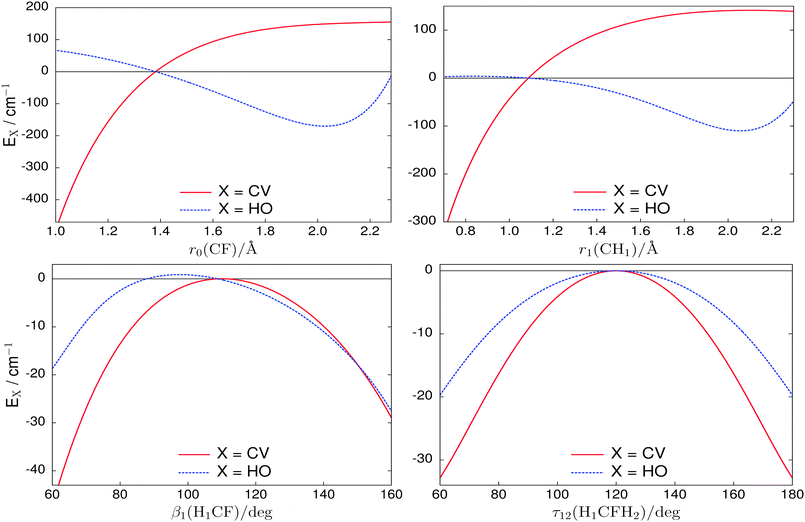Fig. 1 One-dimensional cuts of the core-valence (CV) and higher-order (HO) corrections with all other coordinates held at their equilibrium values.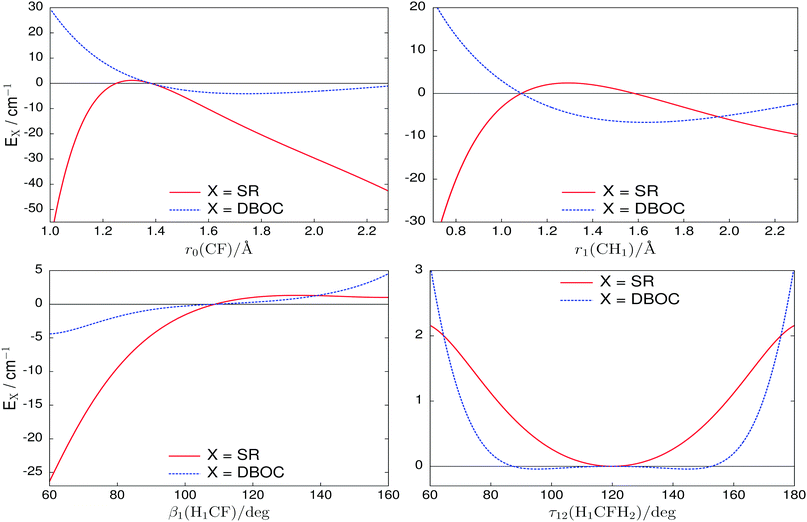Fig. 2 One-dimensional cuts of the scalar relativistic (SR) and diagonal Born–Oppenheimer (DBOC) corrections with all other coordinates held at their equilibrium values.

### 2.2 Analytic representation

Methyl fluoride is a prolate symmetric top molecule of C3v(M) molecular symmetry.48 The XY3Z symmetrized analytic representation utilized in this work has previously been employed for nuclear motion calculations of CH3Cl.15 Morse oscillator functions describe the stretching coordinates,
 ξ1 = 1 − exp[−a(r0 − rref0)], (3)
 ξj = 1 − exp[−b(ri − rref1)]; j = 2, 3, 4, i = j − 1, (4)
where a = 1.90 Å−1 for the C–F internal coordinate r0, and b = 1.87 Å−1 for the three C–H internal coordinates r1, r2 and r3. For the angular terms,
 ξk = (βi − βref); k = 5, 6, 7, i = k − 4, (5)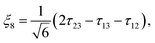(6)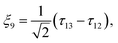(7)
where τ23 = 2π − τ12τ13, and rref0, rref1 and βref are the reference equilibrium structural parameters. Values of rref0 = 1.3813 Å, rref1 = 1.0869 Å, and βref = 108.773° have been used, however, this choice is somewhat arbitrary due to the inclusion of linear expansion terms in the parameter set of the PES. Thus, the reference equilibrium structural parameters do not define the minimum of the PES and the true equilibrium values will be discussed in Section 5.1.

The potential function,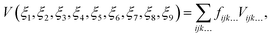(8)
has maximum expansion order i + j + k + l + m + n + p + q + r = 6 and is composed of the terms
 Vijk… = {ξi1ξj2ξk3ξl4ξm5ξn6ξp7ξq8ξr9}C3v(M), (9)
which are symmetrized combinations of different permutations of the coordinates ξi that transform according to the A1 representation of C3v(M). The terms in eqn (9) are determined on-the-fly during the variational calculations and the PES implementation requires only a small amount of code.15

To determine the expansion parameters fijk in eqn (8), a least-squares fitting to the ab initio data was carried out. Weight factors of the form proposed by Schwenke49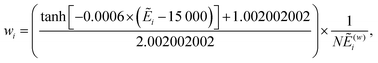(10)
were used in the fit. Here, (w)i = max(i,10000), where i is the potential energy at the ith geometry above equilibrium and the normalization constant N = 0.0001 (all units assume the energy is in cm−1). Energies below 15000 cm−1 are favoured in our fitting by the weighting scheme. Watson's robust fitting scheme50 was also utilized to further improve the description at lower energies and reduce the weights of outliers. The final PES was fitted with a weighted root-mean-square (rms) error of 0.97 cm−1 for energies up to hc·50000 cm−1 and required 405 expansion parameters.

For geometries where r0 ≥ 1.95 Å and ri ≥ 2.10 Å for i = 1, 2, 3, the respective weights were reduced by several orders of magnitude. At larger stretch distances a T1 diagnostic value >0.02 indicates that the coupled cluster method has become unreliable.51 Despite energies not being wholly accurate at these points, they are still useful and ensure that the PES maintains a reasonable shape towards dissociation. In subsequent calculations we refer to the PES as CBS-F12HL. The expansion parameters and a Fortran routine to construct the CBS-F12HL PES are provided in the ESI.

## 3 Dipole moment surface

### 3.1 Electronic structure calculations

In a Cartesian laboratory-fixed XYZ coordinate system with origin at the C nucleus, an external electric field with components ±0.005 a.u. was applied along each coordinate axis and the respective dipole moment component μA for A = X, Y, Z determined using central finite differences. Calculations were carried out in MOLPRO201236 at the CCSD(T)-F12b/aug-cc-pVTZ level of theory and employed the frozen core approximation with a Slater geminal exponent value of β = 1.2a0−1.32 The same ansatz and ABS as in the explicitly correlated PES calculations were used. The DMS was computed on the same grid of nuclear geometries as the PES.

### 3.2 Analytic representation

The analytic representation used for the DMS of methyl fluoride was previously employed for CH3Cl and the reader is referred to Owens et al.52 for a detailed description. To begin with, it is necessary to transform to a suitable molecule-fixed xyz coordinate system before fitting an analytic expression to the ab initio data. A unit vector is defined along each bond of CH3F,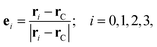(11)
where rC is the position vector of the C nucleus, r0 the F nucleus, and r1, r2 and r3 the respective H atoms. The ab initio dipole moment vector μ is projected onto the molecular bonds and is described by three molecule-fixed xyz dipole moment components,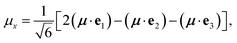(12)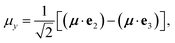(13)
 μz = μ·e0. (14)
We have formed symmetry-adapted combinations for μx and μy which transform according to the E representation of C3v(M), while the μz component is of A1 symmetry. The symmetrized molecular bond representation described here is beneficial as the unit vectors ei that define μ for any instantaneous configuration of the nuclei are related to the internal coordinates only, meaning the description is self-contained.

The three dipole moment surfaces μα for α = x, y, z corresponding to eqn (12)–(14) are represented by the analytic expression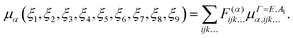(15)
The expansion terms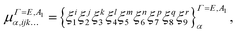(16)
have maximum expansion order i + j + k + l + m + n + p + q + r = 6 and are best understood as a sum of symmetrized combinations of different permutations of the coordinates ξi. Note that Γ = E for μx and μy, and Γ = A1 for μz. For the stretching coordinates we employed linear expansion variables,
 ξ1 = (r0 − rref0), (17)
 ξj = (ri − rref1); j = 2, 3, 4, i = j − 1, (18)
whilst the angular terms are the same as those defined in eqn (5)–(7). The reference structural parameters rref0, rref1 and βref had the same values as in the case of the PES.

The expansion coefficients F(α)ijk for α = x, y, z were determined simultaneously through a least-squares fitting to the ab initio data. Weight factors of the form given in eqn (10) were used along with Watson's robust fitting scheme.50 The three dipole moment surfaces, μx, μy, and μz, required 171, 160 and 226 parameters, respectively. A combined weighted rms error of 1 × 10−4 D was achieved for the fitting. Similar to the PES, the analytic representation of the DMS is generated on-the-fly at runtime. Its construction is slightly more complex because μ is a vector quantity and the transformation properties of the dipole moment components must also be considered.52 The expansion parameter set of the DMS is given in the ESI along with a Fortran routine to construct the analytic representation.

## 4 Variational calculations

The general methodology of TROVE is well documented53–56 and calculations on another XY3Z molecule, namely CH3Cl, have previously been reported.15,52 We therefore summarize only the key aspects relevant for this work.

The rovibrational Hamiltonian was represented as a power series expansion around the equilibrium geometry in terms of the nine coordinates introduced in eqn (3)–(7). However, for the kinetic energy operator linear displacement variables (rirref) were used for the stretching coordinates. The Hamiltonian was constructed numerically using an automatic differentiation method55 with both the kinetic and potential energy operators truncated at sixth order. The associated errors of such a scheme are discussed in Yurchenko et al.53 and Yachmenev and Yurchenko.55 Atomic mass values57 were employed throughout.

A multi-step contraction scheme56 was used to construct the symmetrized vibrational basis set, the size of which was controlled by the polyad number,

 P = n1 + 2(n2 + n3 + n4) + n5 + n6 + n7 + n8 + n9 ≤ Pmax, (19)
and this does not exceed a predefined maximum value Pmax. Here, the quantum numbers nk for k = 1,…,9 relate to primitive basis functions ϕnk, which are obtained by solving one-dimensional Schrödinger equations for each kth vibrational mode using the Numerov–Cooley method.58,59 Multiplication with symmetrized rigid-rotor eigenfunctions |J,K,m,τrot〉 produced the final basis set for use in J > 0 calculations. The quantum numbers K and m are the projections (in units of ħ) of J onto the molecule-fixed z axis and the laboratory-fixed Z axis, respectively, whilst τrot determines the rotational parity as (−1)τrot. As shown in Fig. 3, the size of the Hamiltonian matrix, i.e., the number of J = 0 basis functions, grows exponentially with respect to Pmax and TROVE calculations above Pmax = 14 were not possible with the resources available to us. We will see in Section 5 that differently sized basis sets and basis set techniques must be utilized when computing various spectroscopic quantities due to the computational demands of variational calculations.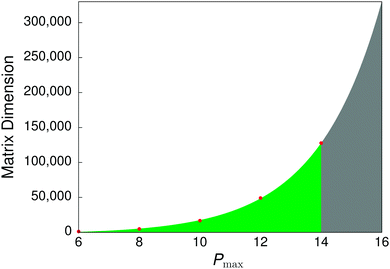Fig. 3 Size of the J = 0 Hamiltonian matrix with respect to the polyad truncation number Pmax. Calculations were not possible above Pmax = 14.

## 5 Results

### 5.1 Equilibrium geometry and pure rotational energies

The equilibrium geometry derived from the CBS-F12HL PES is listed in Table 1. It is in excellent agreement with previous values determined in a joint experimental and ab initio analysis by Demaison et al.,60 which is regarded as the most reliable equilibrium structure of CH3F to date. Also validating is the agreement with the refined geometry PES of Nikitin et al.,24 particularly as the CBS-F12HL PES has been generated in a purely ab initio fashion.
Table 1 The equilibrium structural parameters of CH3F
r(C–F)/Å r(C–H)/Å β(HCF)/deg Ref. Approach
1.38242 1.08698 108.746 This work Purely ab initio PES
1.3826(3) 1.0872(3) 108.69(4) Demaison et al.60 Experimental and ab initio analysis
1.3827 1.0876 108.75 Demaison et al.60 Ab initio calculations
1.38240 1.08696 108.767 Nikitin et al.24 Refined geometry PES

It is more illustrative to look at the pure rotational energy levels, shown in Table 2, since these are highly dependent on the molecular geometry through the moments of inertia. Calculations with TROVE employed a polyad truncation number of Pmax = 8, which is sufficient for converging ground state rotational energies. Despite being consistently lower than the experimental values, the rotational energies up to J ≤ 5 are reproduced with an rms error of 0.0015 cm−1. The residual error ΔE(obs − calc) increases at each step up in J, however, this can be easily counteracted by refining the equilibrium geometry of the CBS-F12HL PES through a nonlinear least-squares fitting to the experimental energies, for example, see Owens et al.17 The accuracy of the computed intra-band rotational wavenumbers can be substantially improved as a result, but we refrain from doing this here as the errors for CH3F are very minor and it leads to a poorer description of the vibrational energy levels.

Table 2 Comparison of computed and experimental J ≤ 5 pure rotational term values (in cm−1) for CH3F. The observed ground state energy levels are taken from Nikitin et al.24 but are attributed to Demaison et al.60
J K Sym. Experiment Calculated Obs − calc
0 0 A 1 0.00000 0.00000 0.00000
1 0 A 2 1.70358 1.70348 0.00010
1 1 E 6.03369 6.03352 0.00017
2 0 A 1 5.11069 5.11040 0.00029
2 1 E 9.44074 9.44038 0.00036
2 2 E 22.43005 22.42946 0.00059
3 0 A 2 10.22124 10.22066 0.00058
3 1 E 14.55120 14.55055 0.00065
3 2 E 27.54025 27.53937 0.00088
3 3 A 1 49.18585 49.18459 0.00126
3 3 A 2 49.18585 49.18459 0.00126
4 0 A 1 17.03508 17.03411 0.00097
4 1 E 21.36493 21.36388 0.00105
4 2 E 34.35362 34.35235 0.00127
4 3 A 1 55.99863 55.99698 0.00165
4 3 A 2 55.99863 55.99698 0.00165
4 4 E 86.29575 86.29356 0.00219
5 0 A 2 25.55202 25.55057 0.00145
5 1 E 29.88172 29.88019 0.00153
5 2 E 42.86997 42.86822 0.00175
5 3 A 1 64.51425 64.51212 0.00213
5 3 A 2 64.51425 64.51212 0.00213
5 4 E 94.81034 94.80767 0.00267
5 5 E 133.75234 133.74897 0.00337

### 5.2 Vibrational J = 0 energy levels

To assess the CBS-F12HL PES it is necessary to have converged vibrational energy levels, and one method of ensuring this is a complete vibrational basis set (CVBS) extrapolation.61 Similar to basis set extrapolation techniques of electronic structure theory (see e.g. Feller62 and references therein), the same ideas can be applied to TROVE calculations with respect to Pmax. Vibrational energies were computed for Pmax = {10,12,14} and fitted using the exponential decay expression,
 Ei(Pmax) = ECVBSi + Aiexp(−λiPmax), (20)
where Ei is the energy of the ith level, ECVBSi is the respective energy at the CVBS limit, Ai is a fitting parameter, and λi is determined from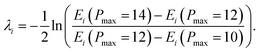(21)

The CVBS extrapolated J = 0 energies are shown in Table 3 alongside known experimental values.63–70 The six fundamentals are reproduced with an rms error of 0.69 cm−1 and a mean-absolute-deviation (mad) of 0.53 cm−1. This level of accuracy extends to most of the other term values which are well within the ±1 cm−1 accuracy expected from PESs based on high-level ab initio theory. Most significant perhaps is the computed ν4 level which shows a residual error ΔE(obs − calc) of −1.26 cm−1. This is a noticeable improvement compared to the PES of Zhao et al.25Eν4 = 3.33 cm−1), which was generated at the CCSD(T)-F12a/aug-cc-pVTZ level of theory, and the PES of Nikitin et al.24Eν4 = 4.86 cm−1), computed at the CCSD(T)/cc-pVQZ level of theory with relativistic corrections, thus highlighting the importance of including HL corrections and a CBS extrapolation in the PES of CH3F.

Table 3 Comparison of computed and experimental J = 0 vibrational term values (in cm−1) for CH3F. The computed zero-point energy was 8560.2409 cm−1 at the CVBS limit
Mode Sym. Experiment Calculated Obs − calc Ref.
ν 3 A 1 1048.61 1048.88 −0.27 63
ν 6 E 1182.67 1182.79 −0.12 63
ν 2 A 1 1459.39 1459.67 −0.28 63
ν 5 E 1467.81 1468.03 −0.21 63
2ν3 A 1 2081.38 2081.81 −0.43 64
ν 3 + ν6 E 2221.81 2222.20 −0.40 64
2ν6 E 2365.80 2365.96 −0.16 65
ν 2 + ν3 A 1 2499.80 2500.28 −0.48 65
ν 3 + ν5 E 2513.80 2514.27 −0.47 65
2ν5 A 1 2863.24 2863.90 −0.66 66
ν 2 + ν5 E 2922.23 2922.59 −0.36 66
2ν2 A 1 2926.00 2926.66 −0.66 66
2ν5 E 2927.39 2927.92 −0.53 66
ν 1 A 1 2966.25 2967.30 −1.05 66
ν 4 E 3005.81 3007.07 −1.26 66
3ν3 A 1 3098.44 3098.97 −0.53 67
ν 3 + 2ν5 A 1 3905.4 3906.39 −0.99 68
ν 1 + ν3 A 1 4011 4012.28 −1.28 69
ν 3 + ν4 E 4057.6 4059.31 −1.71 70
2ν4 E 6000.78 6003.11 −2.33 70

It is worth noting, at least for the values considered in Table 3, that the computed Pmax = 14 vibrational energy levels are within 0.01 cm−1 of the CVBS values with the majority converged to one or two orders-of-magnitude better. A complete list of the Pmax = 14 computed J = 0 energy levels is included in the ESI.

### 5.3 Vibrational transition moments

The vibrational transition moment is defined as,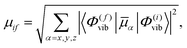(22)
where |Φ(i)vib〉 and |Φ(f)vib〉 are the J = 0 initial and final state eigenfunctions, respectively, and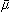α is the electronically averaged dipole moment function along the molecule-fixed axis α = x, y, z. Transition moments are relatively inexpensive to compute and provide an initial assessment of the DMS. Calculations in TROVE used Pmax = 12 and considered transitions from the ground vibrational state only (i = 0).

In Table 4, vibrational transition moments for the fundamentals are listed alongside known experimental values derived from measurements of absolute line intensities.71–73 The agreement is encouraging but it suggests that the DMS may overestimate the strength of line intensities. This behaviour will be confirmed in the following sections. A list of computed transition moments from the vibrational ground state up to 10000 cm−1 is provided in the ESI. For the ground-state electric dipole moment of CH3F we compute a value of 1.8503 D, which is close to the experimentally determined value of 1.8584 D.74

Table 4 Vibrational transition moments (in Debye) for the fundamental frequencies (in cm−1) of CH3F
Mode Sym. ν exp0f μ calc0f μ exp0f Ref.
ν 1 A 1 2966.25 0.05205
ν 2 A 1 1459.39 0.01390 0.01196 73
ν 3 A 1 1048.61 0.20020 0.19015 71
ν 4 E 3005.81 0.08485
ν 5 E 1467.81 0.04903 0.04976 73
ν 6 E 1182.67 0.03085 0.02835 72

### 5.4 Absolute line intensities of the ν6 band

Recently, Jacquemart and Guinet75 generated an experimental line list of almost 1500 transitions of the ν6 band with absolute line intensities determined with an estimated accuracy of 5%. To compare with this study we have generated an ab initio room temperature line list for CH3F. This was computed with a lower state energy threshold of hc·5000 cm−1 and considered transitions up to J = 40 in the 0–4600 cm−1 range.

Describing high rotational excitation can quickly become computationally intractable since rovibrational matrices scale linearly with J. It was therefore necessary to use a truncated J = 0 basis set. TROVE calculations were initially performed with Pmax = 12, resulting in 49076 vibrational basis functions, which was subsequently reduced to 2153 functions by removing states with energies above hc·9600 cm−1. The resulting pruned basis set was multiplied in the usual manner with symmetrized rigid-rotor functions to produce the final basis set for J > 0 calculations.

Naturally, errors are introduced into our rovibrational predictions and it is hard to quantify this without more rigorous calculations. However, we have previously used truncated basis set procedures to construct a comprehensive line list for SiH4 without noticeable deterioration.76 It should be emphasised that the main advantage of truncation is the ability to retain the accuracy of the vibrational energy levels and respective wavefunctions generated with Pmax = 12.

Absolute absorption intensities were simulated using the expression,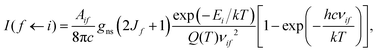(23)
where Aif is the Einstein-A coefficient of a transition with wavenumber νif (in cm−1) between an initial state with energy Ei and a final state with rotational quantum number Jf. Here, k is the Boltzmann constant, h is the Planck constant, c is the speed of light and the absolute temperature T = 296 K. The nuclear spin statistical weights are gns = {8,8,8} for states of symmetry {A1,A2,E}, respectively, and for the room temperature partition function Q(296 K) = 14587.780.27 Transitions obey the symmetry selection rules A1A2, EE; and the standard rotational selection rules, J′ − J′′ = 0, ±1, J′ + J′′ ≠ 0; where ′ and ′′ denote the upper and lower state, respectively. The ExoCross code77 was employed for all spectral simulations.

In Fig. 4, the computed absolute line intensities of the ν6 band are plotted against the experimental line list of Jacquemart and Guinet75 alongside the percentage measure %[(obs − calc)/obs], which quantifies the error in our predicted intensities. The shape and structure of the ν6 band is well reproduced but the DMS overestimates the strength of line intensities. We expect that this behaviour can be corrected for by using a larger augmented basis set in the electronic structure calculations, however, the improvement in intensities may not justify the additional computational expense. As expected from the J = 0 calculations, computed line positions of the ν6 band had an average residual error of Δν(obs − calc) = −0.125 cm−1.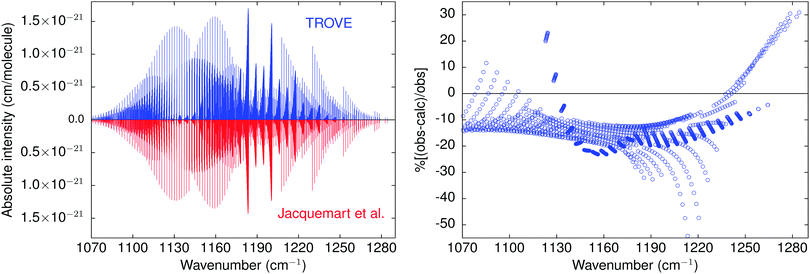Fig. 4 Absolute line intensities of the ν6 band for transitions up to J = 40 at T = 296 K and the corresponding residual errors %[(obs − calc)/obs] when compared with the experimental line list from Jacquemart and Guinet.75

### 5.5 Overview of the rotation–vibration spectrum

A final benchmark of our rovibrational calculations and ab initio line list is a comparison with the PNNL spectral library.78 Absorption cross-sections were simulated at a resolution of 0.06 cm−1 using a Gaussian profile with a half-width at half-maximum of 0.135 cm−1. The results are shown in Fig. 5 and 6 with the experimental PNNL spectrum, which was measured at a temperature of 25 °C with the dataset subsequently re-normalized to 22.84 °C (296 K). Overall the agreement is extremely pleasing, particularly as both the strong and weak intensity features are equally well reproduced. Whilst the intensities of the ab initio spectrum are stronger, this is only slight and we have no hesitation recommending the PES and DMS for future use in spectroscopic applications.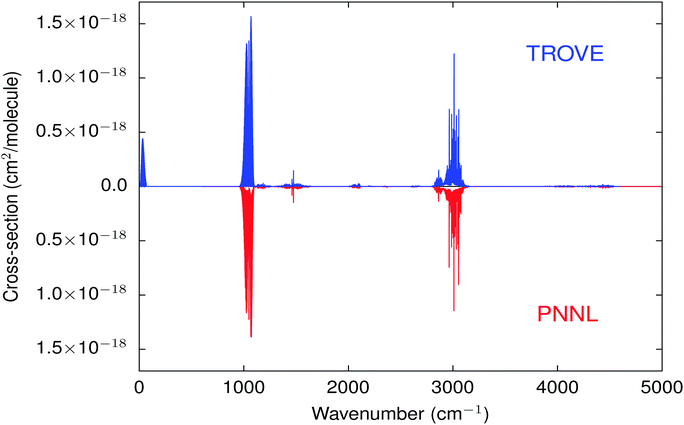Fig. 5 Simulated CH3F rotation–vibration spectrum up to J = 40 compared with the PNNL spectral library78 at T = 296 K.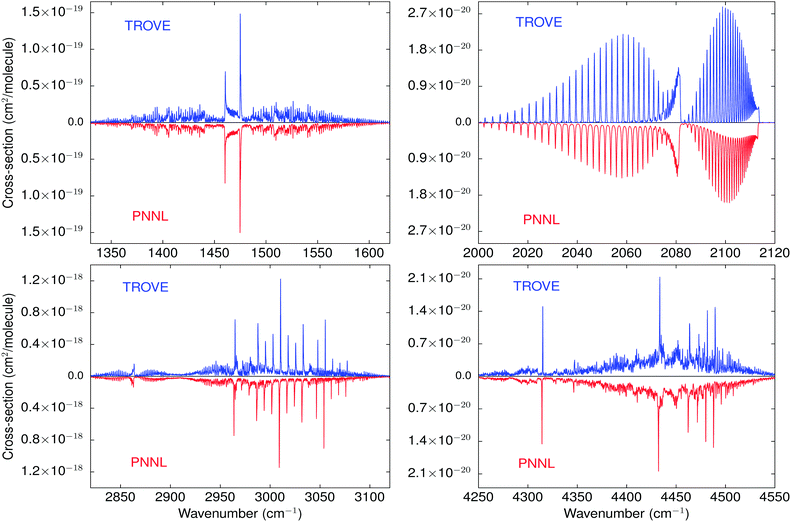Fig. 6 Closer inspection of the computed cross-sections compared with the PNNL spectral library78 at T = 296 K.

## 6 Conclusions

A new PES and DMS for methyl fluoride have been generated using high-level ab initio theory and then rigorously evaluated through variational nuclear motion calculations. The computed results showed excellent agreement with a range of experimental data, which included the equilibrium geometry of CH3F, pure rotational and vibrational energies, vibrational transition moments, absolute line intensities of the ν6 band, and the rotation–vibration spectrum up to J = 40. This work demonstrates the importance of including HL energy corrections and an extrapolation to the CBS limit in the PES to accurately describe the rovibrational spectrum of CH3F from first principles.

To go beyond the accuracy achieved in this work in a purely ab initio manner will require extensive larger basis set electronic structure calculations. That said, the computational cost associated with this is unlikely to correlate with the gain in accuracy and empirical refinement of the PES is recommended instead. Although computationally intensive,79 refinement can lead to orders-of-magnitude improvements in the accuracy of the computed rovibrational energy levels and consequently more reliable transition intensities as a result of better wavefunctions.

## Conflicts of interest

There are no conflicts of interest to declare.

## Acknowledgements

Besides DESY, this work has been supported by the Deutsche Forschungsgemeinschaft (DFG) through the excellence cluster “The Hamburg Center for Ultrafast Imaging – Structure, Dynamics and Control of Matter at the Atomic Scale” (CUI, EXC1074) and the priority program 1840 “Quantum Dynamics in Tailored Intense Fields” (QUTIF, KU1527/3), by the Helmholtz Association “Initiative and Networking Fund”, and by the COST action MOLIM (CM1405).

## References

1. K. M. Dunn, J. E. Boggs and P. Pulay, J. Chem. Phys., 1987, 86, 5088–5093 CrossRef CAS.
2. G. D. Carney, L. L. Sprandel and C. W. Kern, Adv. Chem. Phys., 1978, 37, 305–379 CAS.
3. J. Tennyson, J. Chem. Phys., 2016, 145, 120901 CrossRef PubMed.
4. J. Tennyson and S. N. Yurchenko, Mon. Not. R. Astron. Soc., 2012, 425, 21–33 CrossRef CAS.
5. J. Tennyson, S. N. Yurchenko, A. F. Al-Refaie, E. J. Barton, K. L. Chubb, P. A. Coles, S. Diamantopoulou, M. N. Gorman, C. Hill, A. Z. Lam, L. Lodi, L. K. McKemmish, Y. Na, A. Owens, O. L. Polyansky, T. Rivlin, C. Sousa-Silva, D. S. Underwood, A. Yachmenev and E. Zak, J. Mol. Spectrosc., 2016, 327, 73–94 CrossRef CAS.
6. J. Tennyson and S. N. Yurchenko, Int. J. Quantum Chem., 2017, 117, 92–103 CrossRef CAS.
7. A. Owens, S. N. Yurchenko, W. Thiel and V. Špirko, Mon. Not. R. Astron. Soc., 2015, 450, 3191–3200 CrossRef CAS.
8. A. Owens, S. N. Yurchenko, O. L. Polyansky, R. I. Ovsyannikov, W. Thiel and V. Špirko, Mon. Not. R. Astron. Soc., 2015, 454, 2292–2298 CrossRef CAS.
9. A. Owens, S. N. Yurchenko, W. Thiel and V. S. Špirko, Phys. Rev. A, 2016, 93, 052506 CrossRef.
10. A. Owens, S. N. Yurchenko and V. Špirko, Mon. Not. R. Astron. Soc., 2018, 473, 4986–4992 CrossRef.
11. O. L. Polyansky, A. G. Császár, S. V. Shirin, N. F. Zobov, P. Barletta, J. Tennyson, D. W. Schwenke and P. J. Knowles, Science, 2003, 299, 539–542 CrossRef CAS PubMed.
12. D. W. Schwenke, Spectrochim. Acta, Part A, 2002, 58, 849–861 CrossRef.
13. A. Yachmenev, S. N. Yurchenko, T. Ribeyre and W. Thiel, J. Chem. Phys., 2011, 135, 074302 CrossRef.
14. P. Małyszek and J. Koput, J. Comput. Chem., 2012, 34, 337–345 CrossRef.
15. A. Owens, S. N. Yurchenko, A. Yachmenev, J. Tennyson and W. Thiel, J. Chem. Phys., 2015, 142, 244306 CrossRef PubMed.
16. A. Owens, S. N. Yurchenko, A. Yachmenev and W. Thiel, J. Chem. Phys., 2015, 143, 244317 CrossRef PubMed.
17. A. Owens, S. N. Yurchenko, A. Yachmenev, J. Tennyson and W. Thiel, J. Chem. Phys., 2016, 145, 104305 CrossRef PubMed.
18. T. Helgaker, W. Klopper and D. P. Tew, Mol. Phys., 2008, 106, 2107–2143 CrossRef CAS.
19. K. A. Peterson, D. Feller and D. A. Dixon, Theor. Chem. Acc., 2012, 131, 1079 Search PubMed.
20. S. N. Yurchenko, Chemical Modelling: Volume 10, The Royal Society of Chemistry, 2014, vol. 10, pp. 183–228 Search PubMed.
21. J. Tennyson, J. Mol. Spectrosc., 2014, 298, 1–6 CrossRef CAS.
22. S. A. Manson and M. M. Law, Phys. Chem. Chem. Phys., 2006, 8, 2848–2854 RSC.
23. S. A. Manson, M. M. Law, I. A. Atkinson and G. A. Thomson, Phys. Chem. Chem. Phys., 2006, 8, 2855–2865 RSC.
24. A. V. Nikitin, M. Rey and V. G. Tyuterev, J. Mol. Spectrosc., 2012, 274, 28–34 CrossRef CAS.
25. Z. Zhao, J. Chen, Z. Zhang, D. H. Zhang, D. Lauvergnat and F. Gatti, J. Chem. Phys., 2016, 144, 204302 CrossRef.
26. Z. Zhao, J. Chen, Z. Zhang, D. H. Zhang, X.-G. Wang, T. Carrington and F. Gatti, J. Chem. Phys., 2018, 148, 074113 CrossRef.
27. M. Rey, A. V. Nikitin, Y. L. Babikov and V. G. Tyuterev, J. Mol. Spectrosc., 2016, 327, 138–158 CrossRef CAS.
28. A. G. Császár, W. D. Allen and H. F. Schaefer III, J. Chem. Phys., 1998, 108, 9751–9764 CrossRef.
29. T. B. Adler, G. Knizia and H.-J. Werner, J. Chem. Phys., 2007, 127, 221106 CrossRef PubMed.
30. K. A. Peterson, T. B. Adler and H.-J. Werner, J. Chem. Phys., 2008, 128, 084102 CrossRef.
31. S. Ten-No, Chem. Phys. Lett., 2004, 398, 56–61 CrossRef CAS.
32. J. G. Hill, K. A. Peterson, G. Knizia and H.-J. Werner, J. Chem. Phys., 2009, 131, 194105 CrossRef.
33. K. E. Yousaf and K. A. Peterson, J. Chem. Phys., 2008, 129, 184108 CrossRef.
34. F. Weigend, Phys. Chem. Chem. Phys., 2002, 4, 4285–4291 RSC.
35. C. Hättig, Phys. Chem. Chem. Phys., 2005, 7, 59–66 RSC.
36. H.-J. Werner, P. J. Knowles, G. Knizia, F. R. Manby and M. Schütz, WIREs Comput. Mol. Sci., 2012, 2, 242–253 CrossRef CAS.
37. J. G. Hill, S. Mazumder and K. A. Peterson, J. Chem. Phys., 2010, 132, 054108 CrossRef.
38. M. Kállay and J. Gauss, J. Chem. Phys., 2005, 123, 214105 CrossRef.
39. M. Kállay and J. Gauss, J. Chem. Phys., 2008, 129, 144101 CrossRef.
40. A string-based quantum chemical program suite written by M. Kállay, See also M. Kállay and P. R. Surján, J. Chem. Phys., 2001, 115, 2945 CrossRef as well as http://www.mrcc.hu.
41. Coupled-Cluster techniques for Computational Chemistry, a quantum chemical program package written by J. F. Stanton, J. Gauss, M. E. Harding, and P. G. Szalay with contributions from A. A. Auer, R. J. Bartlett, U. Benedikt, C. Berger, D. E. Bernholdt, Y. J. Bomble, L. Cheng, O. Christiansen, M. Heckert, O. Heun, C. Huber, T.-C. Jagau, D. Jonsson, J. Jusélius, K. Klein, W. J. Lauderdale, D. A. Matthews, T. Metzroth, L. A. Mück, D. P. O'Neill, D. R. Price, E. Prochnow, C. Puzzarini, K. Ruud, F. Schiffmann, W. Schwalbach, S. Stopkowicz, A. Tajti, J. Vázquez, F. Wang, J. D. Watts, and the integral packages MOLECULE (J. Almlöf and P. R. Taylor), PROPS (P. R. Taylor), ABACUS (T. Helgaker, H. J. Aa. Jensen, P. Jørgensen, and J. Olsen), and ECP routines by A. V. Mitin and C. van Wüllen. For the current version, see http://www.cfour.de.
42. T. H. Dunning, J. Chem. Phys., 1989, 90, 1007 CrossRef CAS.
43. M. Douglas and N. M. Kroll, Ann. Phys., 1974, 82, 89–155 CAS.
44. B. A. Heß, Phys. Rev. A: At., Mol., Opt. Phys., 1986, 33, 3742–3748 CrossRef.
45. W. A. de Jong, R. J. Harrison and D. A. Dixon, J. Chem. Phys., 2001, 114, 48–53 CrossRef CAS.
46. G. Tarczay, A. G. Császár, W. Klopper and H. M. Quiney, Mol. Phys., 2001, 99, 1769–1794 CrossRef CAS.
47. J. Gauss, A. Tajti, M. Kállay, J. F. Stanton and P. G. Szalay, J. Chem. Phys., 2006, 125, 144111 CrossRef.
48. P. R. Bunker and P. Jensen, Molecular Symmetry and Spectroscopy, NRC Research Press, Ottawa, Ontario, Canada, 2nd edn, 1998 Search PubMed.
49. H. Partridge and D. W. Schwenke, J. Chem. Phys., 1997, 106, 4618–4639 CrossRef CAS.
50. J. K. G. Watson, J. Mol. Spectrosc., 2003, 219, 326–328 CrossRef CAS.
51. T. J. Lee and P. R. Taylor, Int. J. Quantum Chem., 1989, 36, 199–207 CrossRef.
52. A. Owens, S. N. Yurchenko, A. Yachmenev, J. Tennyson and W. Thiel, J. Quant. Spectrosc. Radiat. Transfer, 2016, 184, 100–110 CrossRef CAS.
53. S. N. Yurchenko, W. Thiel and P. Jensen, J. Mol. Spectrosc., 2007, 245, 126–140 CrossRef CAS.
54. S. N. Yurchenko, R. J. Barber, A. Yachmenev, W. Thiel, P. Jensen and J. Tennyson, J. Phys. Chem. A, 2009, 113, 11845–11855 CrossRef CAS.
55. A. Yachmenev and S. N. Yurchenko, J. Chem. Phys., 2015, 143, 014105 CrossRef.
56. S. N. Yurchenko, A. Yachmenev and R. I. Ovsyannikov, J. Chem. Theory Comput., 2017, 13, 4368 CrossRef CAS PubMed.
57. E. R. Cohen, T. Cvitaš, J. G. Frey, B. Holmström, K. Kuchitsu, R. Marquardt, I. Mills, F. Pavese, M. Quack, J. Stohner, H. L. Strauss, M. Takami and A. J. Thor, Quantities, Units and Symbols in Physical Chemistry, IUPAC & RSC Publishing, Cambridge, 3rd edn, 2008 Search PubMed.
58. B. V. Noumerov, Mon. Not. R. Astron. Soc., 1924, 84, 592–602 CrossRef.
59. J. W. Cooley, Math. Comput., 1961, 15, 363–374 Search PubMed.
60. J. Demaison, J. Breidung, W. Thiel and D. Papoušek, Struct. Chem., 1999, 10, 129–133 CrossRef CAS.
61. R. I. Ovsyannikov, W. Thiel, S. N. Yurchenko, M. Carvajal and P. Jensen, J. Chem. Phys., 2008, 129, 044309 CrossRef.
62. D. Feller, J. Chem. Phys., 2013, 138, 074103 CrossRef.
63. D. Papoušek, P. Pracna, M. Winnewisser, S. Klee and J. Demaison, J. Mol. Spectrosc., 1999, 196, 319–323 CrossRef PubMed.
64. D. Papoušek, J. F. Ogilvie, S. Civiš and M. Winnewisser, J. Mol. Spectrosc., 1991, 149, 109–124 CrossRef.
65. M. Betrencourt and M. Morillon-Chapey, Mol. Phys., 1977, 33, 83–94 CrossRef CAS.
66. J. P. Champion, A. G. Robiette, I. M. Mills and G. Graner, J. Mol. Spectrosc., 1982, 96, 422–441 CrossRef CAS.
67. G. Graner, J. Phys. Chem., 1979, 83, 1491–1499 CrossRef CAS.
68. S. Kondo, Y. Koga and T. Nakanaga, J. Phys. Chem., 1986, 90, 1519–1524 CrossRef CAS.
69. W. E. Blass and T. H. Edwards, J. Mol. Spectrosc., 1968, 25, 440–450 CrossRef.
70. E. W. Jones, R. J. L. Popplewell and H. W. Thompson, Proc. R. Soc. London, Ser. A, 1966, 290, 490–507 CrossRef CAS.
71. M. Lepère, G. Blanquet and J. Walrand, J. Mol. Spectrosc., 1996, 177, 307–310 CrossRef.
72. M. Lepère, G. Blanquet, J. Walrand and J. P. Bouanich, J. Mol. Spectrosc., 1996, 180, 218–226 CrossRef.
73. M. Lepère, G. Blanquet, J. Walrand and G. Tarrago, J. Mol. Spectrosc., 1998, 189, 137–143 CrossRef.
74. M. D. Marshall and J. S. Muenter, J. Mol. Spectrosc., 1980, 83, 279–282 CrossRef CAS.
75. D. Jacquemart and M. Guinet, J. Quant. Spectrosc. Radiat. Transfer, 2016, 185, 58–69 CrossRef CAS.
76. A. Owens, A. Yachmenev, W. Thiel, J. Tennyson and S. N. Yurchenko, Mon. Not. R. Astron. Soc., 2017, 471, 5025–5032 CrossRef CAS.
77. S. N. Yurchenko, A. F. Al-Refaie and J. Tennyson, Astron. Astrophys., 2018 DOI:10.1051/0004-6361/201732531.
78. S. W. Sharpe, T. J. Johnson, R. L. Sams, P. M. Chu, G. C. Rhoderick and P. A. Johnson, Appl. Spectrosc., 2004, 58, 1452–1461 CrossRef CAS.
79. S. N. Yurchenko, R. J. Barber, J. Tennyson, W. Thiel and P. Jensen, J. Mol. Spectrosc., 2011, 268, 123–129 CrossRef CAS.

### Footnote

Electronic supplementary information (ESI) available: The expansion parameters and Fortran 90 functions to construct the PES and DMS of CH3F. A list of computed vibrational energy levels and vibrational transition moments. See DOI: 10.1039/c8cp01721b
 This journal is © the Owner Societies 2019